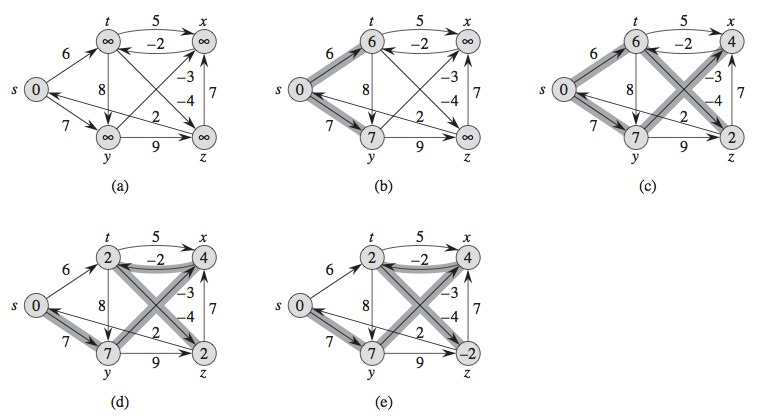# Analysis and Design of Algorithms

July 17, 2019

## Problem of Finding the Shortest Path

• Chapter 24 of Introduction to Algorithms (3rd Edition), Thomas H. Cormen, Charles E. Leiserson, Ronald L. Rivest, and Clifford Stein.

## Problem of Finding the Shortest Path

• We require to get from city $$A$$ to city $$B$$
• We have a map with the distances between each pair of intersections
• How can we find the shortest path?
• Ennumerate all paths from $$A$$ to $$B$$
• Compute the distance
• Choose the shortest
• Even if we remove cycles, there are many possibilities
• We need to solve the problem efficiently

## Problem of Finding the Shortest Path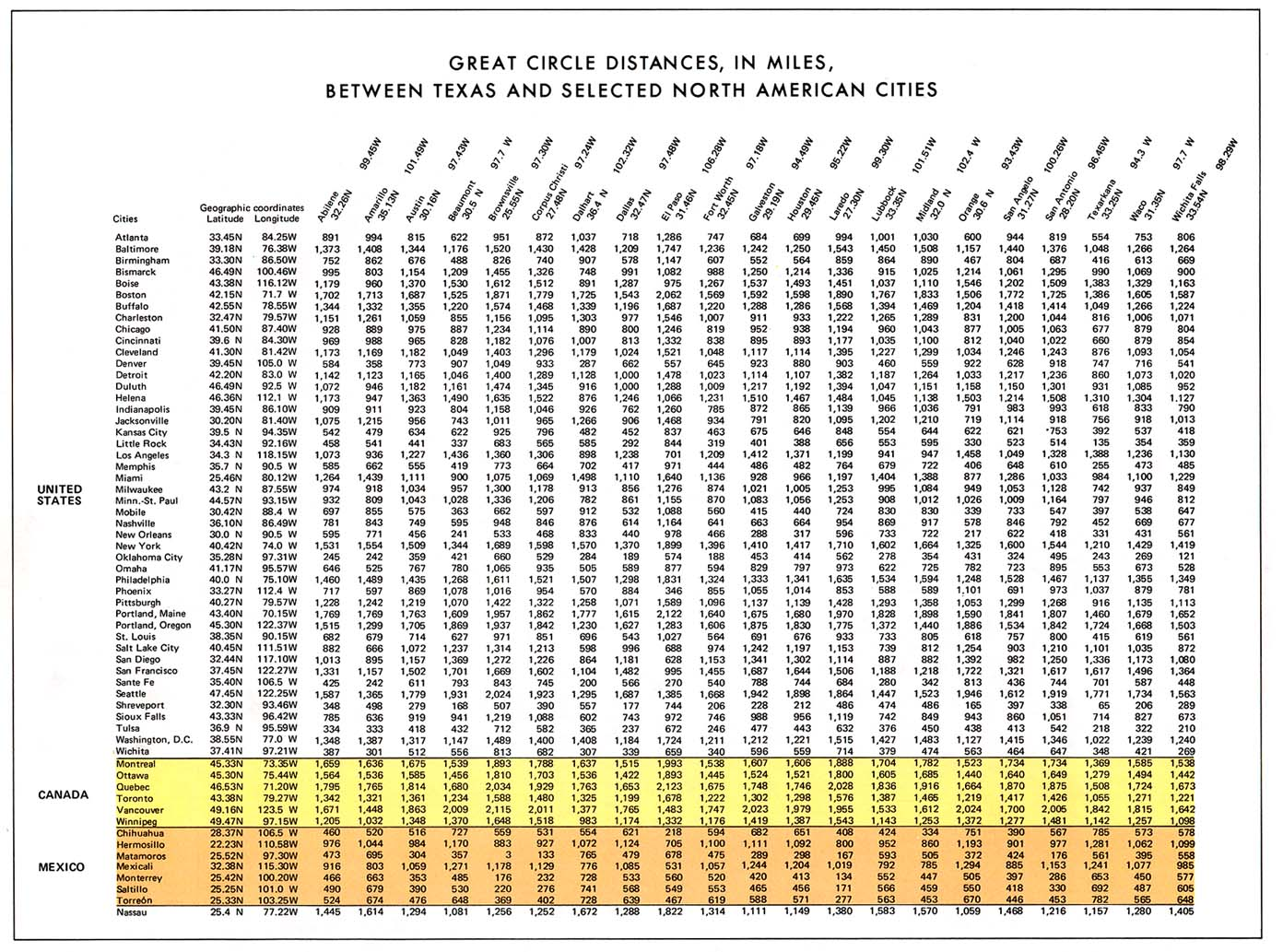## Problem of Finding the Shortest Path

• We have a directed and weighted graph $$G = (V, E)$$
• With a weights function $$w: E \rightarrow R$$ that maps edges $$e$$ to weights with real values
• The weight of the path $$p = \langle v_0, v_1, ..., v_k \rangle$$ is the sum of the weights of the edges contained in it:

$w(p) = \sum_{i = 1}^k w(v_{i-1}, v_i)$

## Problem of Finding the Shortest Path

• We define the weight of the shortest path from $$u$$ to $$v$$ as

$$\delta (u, v) = \begin{cases} min\lbrace w(p): u \overset{p}{\longrightarrow} v\rbrace &\mbox{if there is a path from u to v}\\ \infty &\mbox{any other way}\\ \end{cases}$$

• A Shortest-Path from a vertex $$v$$ to a vertex $$u$$ is defined as any path $$p$$ with weight $$w(p) = \delta(u, v)$$

## Modeling the Problem

• We can model the problem with a graph
• Vertices represent intersections
• Edges represent road segments between intersections
• Weights of edges represent the distances on roads
• The goal consists on finding the shortest path from a city $$A$$ to a city $$B$$
• Weights can also represent other measures
• Time, cost, loses, penalties or any other quantity that linearly accumulates along the path and we want to minimize

## Variants of the Problem

• Shortest paths from a single source
• Given a graph $$G = (V, E)$$, we want to find the shortest path from a given source vertex $$s \in V$$ to each vertex $$v \in V$$.
• Shortest paths with a single target
• Find a shortest path to a given target vertex $$t$$ from each vertex $$v$$. If we change the direction of each edge in the graph, we can reduce this problem to that of a single source

## Variants of the Problem

• Shortest path for a single pair
• Find the shortest path from $$u$$ to $$v$$ for given vertices $$u$$ and $$v$$
• If we solve the single source problem with vertex $$u$$ as single source, we also solve this one
• There is not a known algorithm to solve this problem that runs asymptotically faster than the best algorithm for a single source in the worst case

## Variants of the Problem

• Shortest paths for all pairs
• Find the shortest path from $$u$$ to $$v$$ for each pair of vertices $$u$$ and $$v$$
• Even when this problem can be solved by executing a single source algorithm once for each vertex, it can be solved faster

## Optimal Substructure of a Shortest Path

• Shortest paths algorithms are based in the property that a shortest path between two vertices contains another shortest path in it
• This property allows applying
• Dynamic programming (Floyd-Warshall)
• Greedy algorithms (Dijkstra’s)

## Optimal Substructure of a Shortest Path

• Lemma 24.1 (Subpaths of shortest paths are shortest paths)
• Given a directed and weighted graph $$G = (V, E)$$ with a weight function $$w: E \rightarrow R$$
• Let $$p = \langle v_1, v_2, ..., v_k \rangle$$ a shortest path from vertex $$v_1$$ to vertex $$v_k$$, and
• For any $$i$$ and $$j$$ such that $$1 \leq i \leq j \leq k$$, let $$p_{ij} = \langle v_i, v_{i+1}, ..., v_j \rangle$$, the subpath of $$p$$ from vertex $$v_i$$ to vertex $$v_j$$
• Then $$p_{ij}$$ is a shortest path from $$v_i$$ to $$v_j$$

## Optimal Substructure of a Shortest Path - Proof to lemma 24.1

• Decompose path $$p$$ in $$v_1 \overset{p_{1i}}{\longrightarrow} v_i \overset{p_{ij}}{\longrightarrow} v_j \overset{p_{jk}}{\longrightarrow} v_k$$
• We then have that $$w(p) = w(p_{1i}) + w(p_{ij}) + w(p_{jk})$$
• We assume that there is a path $$p_{ij}\prime$$ from $$v_i$$ to $$v_j$$ with weight $$w(p_{ij}^\prime) \le w(p_{ij})$$
• Then the path from $$v_1$$ to $$v_k$$ that passes through $$p_{ij}^\prime$$ $$v_1 \overset{p_{1i}}{\longrightarrow} v_i \overset{p_{ij}^\prime}{\longrightarrow} v_j \overset{p_{jk}}{\longrightarrow} v_k$$ with weight $$w(p_{1i}) + w(p_{ij}^\prime) + w(p_{jk})$$ has a weight lower than $$w(p)$$
• This is a contradiction to our assumption, that $$p$$ is a shortest path from $$v_1$$ to $$v_k$$

## Representation of the Shortest paths

• We use a predecessors graph $$G_\pi = (V_\pi, E_\pi)$$
• $$\pi[v]$$ denotes the father of vertex $$v$$
• At the end, the predecessors graph is a tree of shortest paths
• A tree with root that contains a shortest path with a source $$s$$ to each vertex that is reachable from $$s$$
• A shortest paths tree with root $$s$$ is a directed subgraph $$G^\prime = (V^\prime, E^\prime)$$, where $$V^\prime \subseteq V$$ and $$E^\prime \subseteq E$$ such that
• $$V^\prime$$ is the set of reachable vertices from $$s$$ in $$G$$
• $$G^\prime$$ forms a tree with root $$s$$ and
• For each $$v \in V^\prime$$, the only simple path from $$s$$ to $$v$$ in $$G^\prime$$ is a shortest path from $$s$$ to $$v$$ in $$G$$

## Representation of the Shortest paths

• Shortest paths are not necessarily unique
• Two shortest paths trees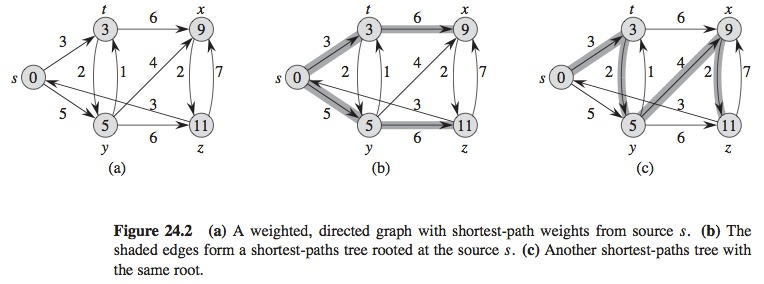## Relaxation Technique

• These algorithms use the relaxation technique
• For each vertex $$v \in V$$, we keep an attribute $$d[v]$$
• An upper threshold of the weight of the shortest path from the source $$s$$ to $$v$$
• $$d[v]$$ is called an estimate of the shortest path

## Relaxation Technique

• Initialization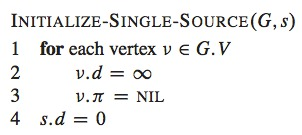## Relaxation Technique

• The relaxation of an edge $$(u, v)$$ consists on
• Test if we can enhance the shortest path to $$v$$ found through $$u$$, and if possible, update $$d[v]$$ and $$\pi[v]$$
• The process may decrease the value of the estimation of the shortest path $$d[v]$$ and update predecessor $$\pi[v]$$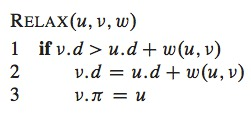## Relaxation Technique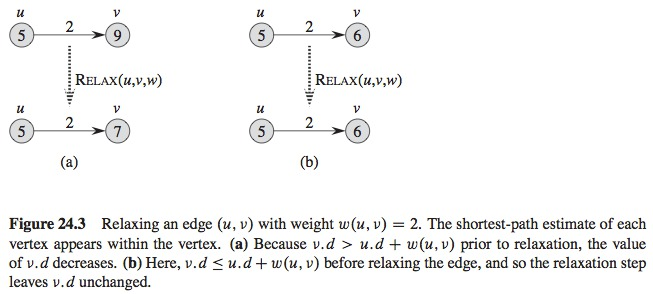## Relaxation Technique

• Lemmas 24.10, 24.11, 24.14, 24.15, and 24.17 and corolary 24.12 show how by relaxing after INITIALIZE-SINGLE-SOURCE, the shortest path weight will be reached and the predecessors graph will be a tree of shortest paths
• We assume that there are no cycles with negative weight

## Negative Cycles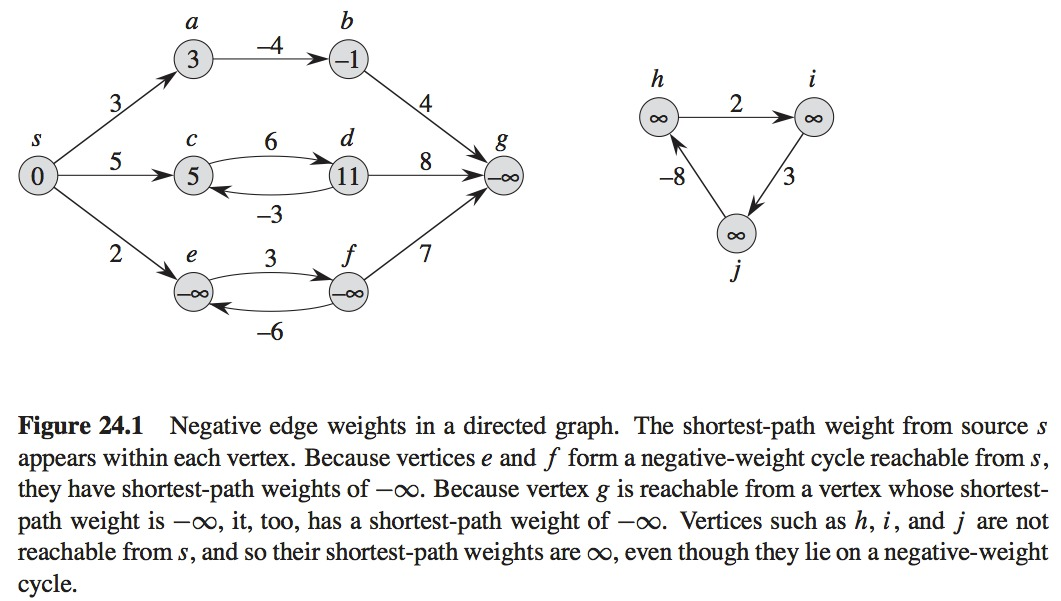## Properties of Shortest Paths and Relaxation

• Triangle Inequality (Lemma 24.10)
• For any edge $$(u, v) \in E$$, we have $$\delta(s, v) \leq \delta(s, u) + w(u, v)$$.
• Upper-bound Property (Lemma 24.11)
• We always have $$v.d \geq \delta(s, v)$$ for all vertices $$v \in V$$, and once $$v.d$$ achieves the value $$\delta(s, v)$$, it never changes.

## Properties of Shortest Paths and Relaxation

• No-path Property (Corollary 24.12)
• If there is no path from $$s$$ to $$v$$, then we always have $$v.d = \delta(s, v) = \infty$$.
• Convergence Property (Lemma 24.14)
• If $$s \leadsto u \rightarrow v$$ is a shortest path in $$G$$ for some $$u$$, $$v \in V$$, and if $$u.d = \delta(s, u)$$ at any time prior to relaxing edge $$(u, v)$$, then $$v.d = \delta(s, v)$$ at all times afterward.

## Properties of Shortest Paths and Relaxation

• Path-relaxation Property (Lemma 24.15)
• If $$p = \langle v_0, v_1, \dots, v_k \rangle$$ is a shortest path from $$s = v_0$$ to $$v_k$$, and we relax the edges of $$p$$ in the order $$(v_0, v_1), (v_1, v_2), \dots, (v_{k-1}, v_k)$$, then $$v_k.d = \delta(s, v_k)$$.
• This property holds regardless of any other relaxation steps that occur, even if they are intermixed with relaxations of the edges of $$p$$.
• Predecessor-subgraph Property (Lemma 24.17)
• Once $$v.d = \delta(s, v)$$ for all $$v \in V$$, the predecessor subgraph is a shortest-paths tree rooted at $$s$$.

## Bellman-Ford Algorithm

• Solves the shortest paths problem from a single source
• In the general case, there might be edges with negative weights
• Given a directed graph, with weights, with source $$s$$ and weights function $$w: E \rightarrow R$$, returns a boolean value indicating whether there is a cycle with negative weight that is reachable from the source
• If there exists a cycle of this type, the algorithms indicates that there is no solution
• If there is not a cycle of this type, the algorithm produces the shortest paths and their weights

## Bellman-Ford Algorithm

• This algorithm uses the relaxation process
• Progressively decreases an estimate $$d[v]$$ over the weight fo a shortest path from the source $$s$$ to each vertex $$v \in V$$ until it reaches the real weight of the shortest path $$\delta(s, v)$$

## Bellman-Ford Algorithm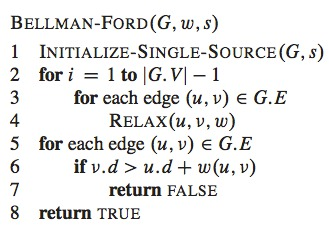## Bellman-Ford Algorithm

• First, initializes, line 1
• Makes $$|V| - 1$$ passes over the edges, loop in lines 2 - 4
• Relaxes each edge of the graph once
• Verifies if there is a cycle with negative weight, lines 5 - 8
• If there is a cycle, returns FALSE
• If there is not a cycle, returns TRUE
• Execution time
• $$O(VE)$$
• Initialization $$\theta(V)$$
• Each pass ($$V - 1$$ in total) because of lines 2 - 4, $$\theta(E)$$
• Cycle in lines 5 - 7, $$O(E)$$

## Bellman-Ford Algorithm